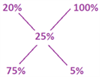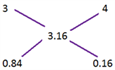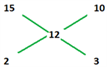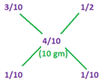# Miscellaneous Arithmetic- Solutions

Check the detailed solutions for Miscellaneous Arithmetic Questions here.

1) option (d)

(Liquid A left after nth operation)/(Initial quantity of A in the vessel) = (a-b)n/an

Amount of phenyl remaining = (1-1/3)4 =16/81

Therefore, ratio or phenyl:water = 16:65. (81-16=65)

2) option (c)

We need to find out how much of a solution of 100% water needs to be added to a solution containing 20% water to attain a dilution of 25%.

This can be found out as followsRatio = 75:5 = 15:1

That is for 15 parts of a 20% water solution, one part of 100% water solution needs to be added.

Therefore, for a solution of 125 litres, 125/15= 8.33 litres need to be added.

3) option (d)

The answer is based on (100-25)3= 753. This translates to 42.18%

4) option (b)

Using alligation, we can directly solve this question Take either petrol or kerosene.

Petrol in cask 1 = 7/10 Petrol in cask 2 = 3/4 Petrol in mixture = 11/15Ratio to take the mixture = 1/60:1/30= 1:2

5) option (b)

This question should be solved using alligation.

For alligation; always consider the cost price only.

Selling Price (SP) Cost Price(CP)

SP of rice 1 = 2.70/kg CP = 2.7/0.9 = 3/kg

SP of rice 2= 4.5/kg CP= 4.5/1.125 = 4/kg

SP of mixture = 3.95/kg CP= 3.95/1.25 = 3.16/kgRequired Ratio = 84:16

6) option (a)

We can infer that the difference between ab and ba is 18. Now all numbers of type 13, 24,35.

I.e. the difference is 2 will give a difference of 18 when reversed. Therefore the difference between a and b will always be 2.

7) Option (c)

Let the present age of each of them be the letters themselves.

The present age of D= C-5

The present age of A will be 2(C-5)+5 =2C-5 and the present age of B will be (C+2C-5)/2 = (3C-5)/2

Average age of members =34

C+C-5+2C-5+ (3C-5)/2=34×4

4C+(3C-5)/2= 146 11C= 297 C=27 D= 27-5=22

8) Option (c)

Using alligationHe should invest in the ratio 2:3.

He should invest 60000

9) option (d)

8 x 50 +R+S-P-Q= 48×8

R+S-P-Q=-16 P+Q-R-S= 16

R=S and P=Q P-R=8

One more person is included and the weight = 48 kg

Let the weight be a (48×8 +a)/9= 48

a=48 kg= weight of R weight of P= 48+8= 56 kg

10) Option (b)

Use Alligation:

Considering only the blue pigment in all the paintsThus, 5 gms of each paint is used. Weight of red paint = 7/10 x 5 =3.5 gms. Option (b)

11) option (d)

To solve this problem quickly, you can come up with likely values for x that would make the mean equal to the median.

Three values that would make the set symmetrical are 0, 10, and 20:

{0, 4, 8, 12, 16} {4, 8, 10, 12, 16}

{4, 8, 12, 16, 20}

To verify if there are any other possibilities for x, we need to evaluate each range of “x”

(1) If x is less than or equal to 8, then the median is equal to 8. We now set the mean equal to the median: (40 + x)/5= 8 40 + x = 40 x = 0

(2) If x is between 8 and 12, then the median is equal to x. (40 + x)/5= x 40 + x = 5x 40 = 4x x = 10

(3) If x is greater than 12, then the median is equal to 12. (40 + x)/5= 12 40 + x = 60 x = 20

12) Option (b)

Let x, y, z be the ages of 3 persons which lies between 60 and 65 The equation governing the problem (55n-41-47+(x+y+z))/(n+1)=56

Also given n is a multiple of 7 55n – 88 +k = 56n + 56 where k =x+y+z, n+ 144=k

Now k ranges between 3×61 and 3×64 183 ≤n +44 ≤ 192 39 ≤n ≤4 Thus n =42

The latest number n + 1 =42+1=43

Shortcut!

Go from answer options. The initial number of people in the group is a multiple of 7.

The answer options give the number of initial people + 1. Thus the correct answer option is option (b)

13) option (d)

In the first shift, he uses the container 6 times. 45 glasses in 5 rounds and the remaining 6 glasses in the 6th round.

Similarly, he prepared 73 glasses in 9 rounds, and 112 glasses in 13 rounds. Hence, the required average

= total time/ total number of rounds = (4 min⁡18 s + 7 min➜13 s + 12 min➜24 s)/( 6+9+13)=(23 min+55s)/28 = 51 seconds

14) option (c)

If we take the amount of sugar as 3x, then rice = 4x and Wheat = 5x

(ratio = 3:4:5; as rice= 4/5 wheat and sugar = 3/4 rice)

12x= 12➜ x=1 Wheat = 5 kg and Sugar = 3 kg.

Total = 8 kg

15) option (c)

V➜ Value d➜ diameter t➜ thickness

given that ➜ V α d2t ratio of diameters = 4:3 and

thickness = t1:t2

V1/V2 =(16 x t1)/(9 x t2 ) ➜ 4= (16t1)/(9t2 ) ➜ t1:t2= 9:4

16) Option (b)

Let us assume that the maximum marks are 100.

Total marks available =500 Aggregate = 3/5 x 500 = 300

Which is divided in the ratio 3:4:5:6:7 Marks are 36, 48,60, 72 and 84.

Only 2 students get more than 70%

17) Option (b)

The total charge = Fixed component +Variable component

Z= F+kx

Where F and x are fixed 550 =F +k(4.5) 300=F + k(2)

Solving simultaneously, F= 100 and k= 100

Charges for 1 hour = 100 +1(100) =200

Questions 18-19

Solution:

Since 8+3=7+4=11, the safest assumption is that there are 110 students in total.

Then, the table will look like this

<tdstyle=”border: 1px solid #E0DDDD !important;”>80

 BOYS GIRLS LILIES 52 28 80 DAISIES 18 12 30 70 40 110

Question 18 ➜28:18 = 14:9

Question 19➜ 1.2x=48 => x= 40

So the number of boys in lilies will be 5.2 × 40 = 208

So the difference will be 208-48 = 160

20) option (b)

The relationship given:- H α √A

H=K√A

4= K√9 ➜ K=4/3

Height of Arun at 16 years➜ H=4/3× 4 =16/3= 5 feet 4 inches

21) option (d)

Let the weight and height of the tree be ‘w’ and ‘h’ respectively.

Thus, h = aw + bw2 where a and b are constants.

Here,

6 = 40a + 402×b 8 = 50a + 502×b

Hence, a = 11/100 and b = 1/1000 If h = 5, 5 = 11/100×w + 1/1000×w2 Solving, w = 35 approximately.

22) option (b)

Let the bus fare = Rs. x and buffet lunch per head = Rs. y

x + 200y = 165 × 200 …(i)

x + 150y = 170 × 150 …(ii)

Then, x + 100y = 2 × (ii) – (i) = 51000 – 33000 = 18000

Therefore, Cost per head = Rs. 180

23) Option (c)

If the addition of 10 litres of pure milk makes the concentration twice what it was before, then the amount added will be the amount of milk present initially.

Replacement of 20 litres, will be the replacement with two 10 litres, making the concentration thrice of what it was initially.

Therefore, the ratio = 1:3

24) Option (c)

Let the excess of speed over 30 kmph=S

Receipts= R and Expenses=E

Then

R=K1 S And E= K2S2

Also, at 60kmph, R=E Thus, K1=30 K2

We need to maximize R-E= K1S- K2S2=SK2(30-S) S+30-S,

the sum is constant, the product will be maximum when they are equal Thus S=30-S => S=15.

Speed = 30+15= 45

25) option (a)

Put r=0, then if 1 kg of 1% grade is mixed with 1 kg of 2% grade,

we get 2 kg of 1.5%grade Hence p=1, q=1 and r=0.